Mathematical Logic as based on the Theory of Types BY   BERTRAND  RUSSELL Published in: American Journal of Mathematics, vol.30(1908), pp. 222-262 Transcribed into hypertext by Burtzev B.I., Jul., 07, 2003e-mail: bbi-math@narod.ru,   site: http://bbi-math.narod.ru/ IX. Cardinal Numbers. FD.The cardinal number of a class α is defined as the class of all classes similar to α, two classes being similar when there is a one-one relation between them. The class of one-one relations is denoted by 1 --> 1, and defined as follows : 1 --> 1 = R^ { xRy . x'Ry . xRy' .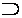x, y, x', y' . x = x' . y = y'}  Df. Similarity is denoted by Sim; its definition is Sim = α^ β^ { (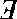R) . R ε 1 --> 1. D*R = α . DinvR = β }  Df. Then Sim----->*α is, by definition, the cardinal number of α; this we will denote by Nc*α; hence we put Nc = Sim----->  Df., whence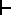. Nc*α = Sim----->*α. The class of cardinals we will denote by NC; thus NC = Nc**cls  Df. 0 is defined as the class whose only member is the null-class, Λ, so that 0 = ι*Λ  Df. The definition of 1 is 1 = α^ { (c) : x ε α .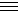x . x = c }  Df. It is easy to prove that 0 and 1 are cardinals according to the definition. FE.It is to be observed, however, that 0 and 1 and all the other cardinals, according to the above definitions, are ambiguous symbols, like cls, and have as many meanings as there are types. To begin with 0: the meaning of 0 depends upon that of Λ, and the meaning of Λ is different according to the type of which it is the null-class. Thus there are as many 0’s as there are types; and the same applies to all the other cardinals. Nevertheless, if two classes α, β are of different types, we can speak of them as having the same cardinal, or of one as having a greater cardinal than the other, because a one-one relation may hold between the members of α and the members of β, even when α and β are of different types. For example, let β be ι**α; i.e., the class whose members are the classes consisting of single members of α. Then ι**α is of higher type than α, but similar to α, being correlated with α by the one-one relation ι. FF.The hierarchy of types has important results in regard to addition. Suppose we have a class of α terms and a class of β terms, where α and β are cardinals; it may be quite impossible to add them together to get a class of α and β terms, since, if the classes are not of the same type, their logical sum is meaningless. Where only a finite number of classes are concerned, we can obviate the practical consequences of this, owing to the fact that we can always apply operations to a class which raise its type to any required extent without altering its cardinal number. For example, given any class α, the class ι**α has the same cardinal number, but is of the next type above α. Hence, given any finite number of classes of different types, we can raise all of them to the type which is what we may call the lowest common multiple of all the types in question; and it can be shown that this can be done in such a way that the resulting classes shall have no common members. We may then form the logical sum of all the classes so obtained, and its cardinal number will be the arithmetical sum of the cardinal numbers of the original classes. But where we have an infinite series of classes of ascending types, this method can not be applied. For this reason, we can not now prove that there must be infinite classes. For suppose there were only n individuals altogether in the uniyerse, where n is finite. There would then be 2n classes of individuals, and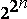classes of classes of individuals, and so on. Thus the cardinal number of terms in each type would be finite; and though these numbers would grow beyond any assigned finite number, there would be no way of adding them so as to get an infinite number. Hence we need an axiom, so it would seem, to the effect that no finite class of individuals contains all individuals; but if any one chooses to assume that the total number of individuals in the universe is (say) 10,367, there seems no a priori way of refuting his opinion. FG.From the above mode of reasoning, it is plain that the doctrine of types avoids all difficulties as to the greatest cardinal. There is a greatest cardinal in each type, namely the cardinal number of the whole of the type ; but this is always surpassed by the cardinal number of the next type, since, if α is the cardinal number of one type, that of the next type is 2α, which, as Cantor has shown, is always greater than α. Since there is no way of adding different types, we can not speak of “the cardinal number of all objects, of whatever type,” and thus there is no absolutely greatest cardinal. FH.If it is admitted that no finite class of individuals contains all individuals, it follows that there are classes of individuals having any finite number. Hence all finite cardinals exist as individual-cardinals; i.e., as the cardinal numbers of classes of individuals. It follows that there is a class of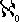cardinals, namely, the class of finite cardinals. Henceexists as the cardinal of a class of classes of classes of individuals. By forming all classes of finite cardinals, we find that 2, exists as the cardinal of a class of classes of classes of classes of indi-dividuals; and so we can proceed indefinitely. The existence of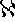n for every finite value of n can also be proved ; but this requires the consideration of ordinals. FI.If, in addition to assuming that no finite class contains all individuals, we assume the multiplicative axiom (i.e., the axiom that, given a set of mutually exclusive classes, none of which are null, there is at least one class consisting of one member from each class in the set), then we can prove that there is a class of individuals containingmembers, so thatexist as an individual-cardinal. This somewhat reduces the type to which we have to go in order to prove the existence-theorem for any given cardinal but it does not give us any existence-theorem which can not be got otherwise sooner or later. FJ.Many elementary theorems concerning cardinals require the multiplicative axiom.26) It is to be observed that this axiom is equivalent to Zermelo's,27) and therefore to the assumption that every class can be well-ordered.28) These equivalent assumptions are, apparently, all incapable of proof, though the multiplicative axiom, at least, appears highly self-evident. In the absence of proof, it seems best not to assume the multiplicative axiom, but to state it as a hypothesis on every occasion on which it is used.
 Notes